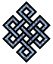#fail2ban bad ip database: ip 212.145.227.246

### | ip database | live view | stats | report | help | api key:

 ip: 212.145.227.246 hostname: static-adsl-b-4-246.ipcom.comunitel.net country:[ES] Spain first reported: 21.11.2018 15:39.04 GMT+0200 last reported: 30.11.2018 04:54.48 GMT+0200 time period: 8d 13h 15m 44s total reports: 11 reported by: 2 host(s) filter(s): ssh (6) ssh (5) tor exit node no badips.com db Lookup## port scan of '212.145.227.246':

[-hide]
```# Nmap 6.40 scan initiated Wed Nov 21 15:40:03 2018 as: /usr/bin/nmap -sU -sS -O 212.145.227.246
Nmap scan report for static-adsl-b-4-246.ipcom.comunitel.net (212.145.227.246)
Host is up (0.032s latency).
Not shown: 1000 open|filtered ports, 989 filtered ports
PORT     STATE  SERVICE
21/tcp   open   ftp
25/tcp   closed smtp
80/tcp   closed http
81/tcp   closed hosts2-ns
113/tcp  closed ident
389/tcp  open   ldap
443/tcp  open   https
1352/tcp closed lotusnotes
1723/tcp open   pptp
2048/tcp closed dls-monitor
3389/tcp open   ms-wbt-server
No exact OS matches for host (If you know what OS is running on it, see http://nmap.org/submit/ ).
TCP/IP fingerprint:
OS:SCAN(V=6.40%E=4%D=11/21%OT=21%CT=25%CU=%PV=N%G=Y%TM=5BF56E66%P=x86_64-pc
OS:-linux-gnu)SEQ(SP=105%GCD=1%ISR=10B%TS=7)OPS(O1=M5A0NW8ST11%O2=M5A0NW8ST
OS:11%O3=M5A0NW8NNT11%O4=M5A0NW8ST11%O5=M5A0NW8ST11%O6=M5A0ST11)WIN(W1=2000
OS:%W2=2000%W3=2000%W4=2000%W5=2000%W6=2000)ECN(R=Y%DF=Y%TG=80%W=2000%O=M5A
OS:0NW8NNS%CC=N%Q=)T1(R=Y%DF=Y%TG=80%S=O%A=S+%F=AS%RD=0%Q=)T2(R=N)T3(R=N)T4
OS:(R=N)T5(R=Y%DF=Y%TG=80%W=0%S=Z%A=S+%F=AR%O=%RD=0%Q=)T6(R=N)T7(R=N)U1(R=N
OS:)IE(R=N)

OS detection performed. Please report any incorrect results at http://nmap.org/submit/ .
# Nmap done at Wed Nov 21 15:40:38 2018 -- 1 IP address (1 host up) scanned in 37.41 seconds
```
```Σ = 41 | Δt = 0.0048129558563232s
```